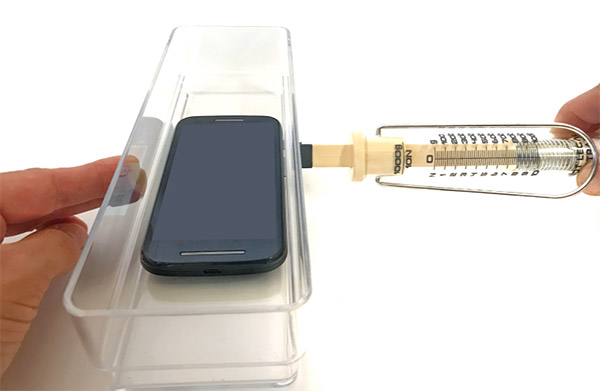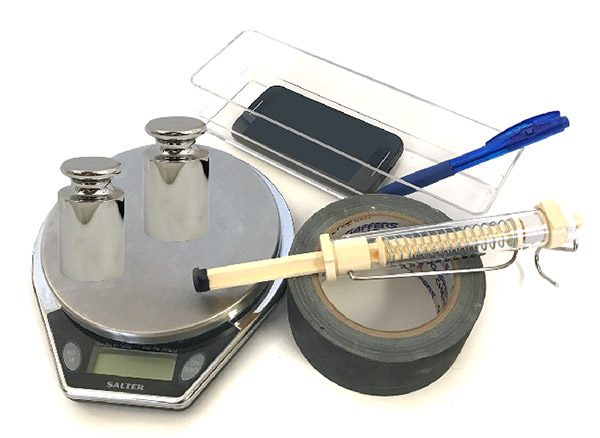# Forces and Motion

9th-12th
Group Size
2-3 students
Active Time
2 hours
Total Time
2 hours
Area of Science
Physics
Key Concepts
Force, mass, acceleration, Newton's second law
Learning Objectives
• Understand the relationship between force, mass, and acceleration as described by Newton's second law of motion.## Overview

Students explore how force, mass, and acceleration are related in this hands-on lesson plan. By experimenting with pushing a box across the table while varying force and mass and measuring the box's acceleration with Google's Science Journal app, students discover Newton's second law of motion for themselves.

## NGSS Alignment

This lesson helps students prepare for these Next Generation Science Standards Performance Expectations:
• HS-PS2-1. Analyze data to support the claim that Newton's second law of motion describes the mathematical relationship among the net force on a macroscopic object, its mass, and its acceleration.
This lesson focuses on these aspects of NGSS Three Dimensional Learning:

 Science & Engineering Practices Disciplinary Core Ideas Crosscutting Concepts Analyzing and Interpreting Data. Analyze data using tools, technologies, and/or models (e.g., computational, mathematical) in order to make valid and reliable scientific claims or determine an optimal design solution. Using Mathematics and Computational Thinking. Use mathematical representations of phenomena to describe explanations. PS2.A: Forces and Motion. Newton's second law accurately predicts changes in the motion of macroscopic objects. Cause and Effect. Empirical evidence is required to differentiate between cause and correlation and make claims about specific causes and effects.

## Credits

Emily Cizmas, Science Teacher
Svenja Lohner, PhD, Science Buddies

## MaterialsMaterials per group of 4 students:

• Push/pull spring scale, available from Amazon.com
• Small plastic box that fits the mobile device. Note: the box should be sturdy and not bend when force is applied with the spring scale. If it deforms, the applied force is not fully transferred to the box, which will affect the data.
• Scale for measuring mass (can be shared by the whole class)
• Weights (2 x 200 grams)
• Tape
• Smartphone or tablet with Google's Science Journal app, available for free on Google Play for Android devices (version 4.4 or newer) or from the App Store for iOS devices (iOS 9.3 or newer).

## Reviews

|
Be the first one to review this lesson plan.
9th-12th
Group Size
2-3 students
Active Time
2 hours
Total Time
2 hours
Area of Science
Physics
Key Concepts
Force, mass, acceleration, Newton's second lawLearning Objectives
• Understand the relationship between force, mass, and acceleration as described by Newton's second law of motion.
Teacher Tool Box